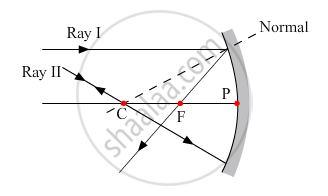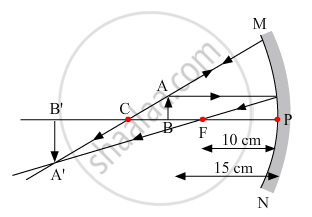Share

# To construct a ray diagram we use two rays of light which are so chosen that it is easy to determine their directions after reflection from the mirror. Choose these two rays and state the path of these rays after reflection from a concave mirror. - CBSE Class 10 - Science

ConceptRepresentation of Images Formed by Spherical Mirrors Using Ray Diagrams - Concave Mirror

#### Question

To construct a ray diagram we use two rays of light which are so chosen that it is easy to determine their directions after reflection from the mirror. Choose these two rays and state the path of these rays after reflection from a concave mirror. Use these two rays to find the nature and position of the image of an object placed at a distance of 15 cm from a concave mirror of focal length 10 cm.

#### Solution

The two rays chosen to construct a ray diagram are shown in the ray diagram given below(i) Ray I: When the incident ray is parallel to the principal axis, the reflected ray will pass through the focus of concave mirror or it appears to pass through the focus of convex mirror.

(ii) Ray II: When the incident ray passes through or appears to pass through the centre of curvature, the light, after reflection from the spherical mirror, reflects back along the same path.The image formed is real, inverted and magnified. It is formed beyond the centre of curvature.

Is there an error in this question or solution?

#### APPEARS IN

Solution To construct a ray diagram we use two rays of light which are so chosen that it is easy to determine their directions after reflection from the mirror. Choose these two rays and state the path of these rays after reflection from a concave mirror. Concept: Representation of Images Formed by Spherical Mirrors Using Ray Diagrams - Concave Mirror.
S